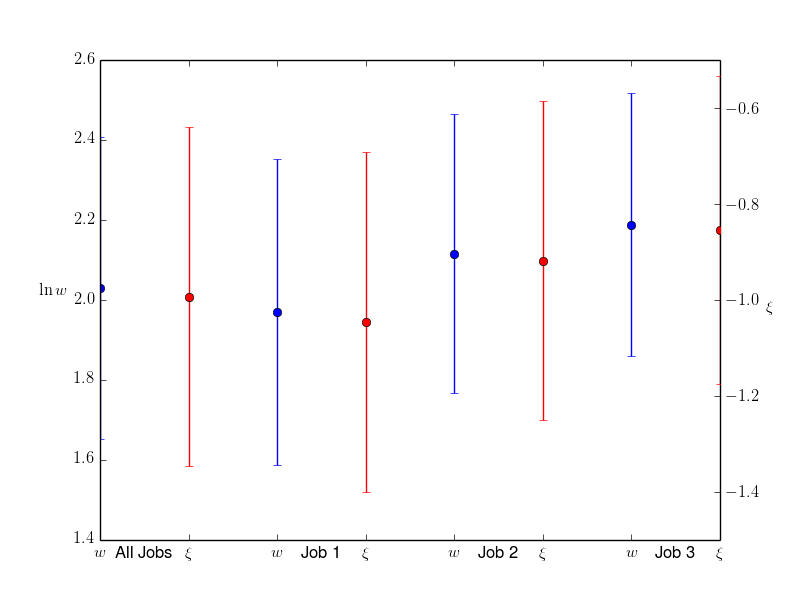# Multiple lines of xtick_labels

Hi,

I'm trying to set two lines of xtick_labels But I can't figure out how
to get them on separate lines. These are for errorbars where I have two
variables for each of four categories. Using the following code, I'm
able to create the attached figure. How does one move the "minor"
xtick_labels to a 2nd line? A minor problem that maybe someone knows
the answer to is, how do I increase the width of the x axis so that
there is some space before and after the first and last ticks?
(ax1.set_xlim(-.5,7.5) does not seem to work.)

Many thanks,
Ted

fig,ax1=plt.subplots()
ax1.errorbar(wageAllTypes.index,wageAllTypes['mean'],yerr=wageAllTypes['sd'],fmt='bo')
xticks=[0,1,2,3,4,5,6,7]
xticks_minor=[.5,2.5,4.5,6.5]
ax1.set_xticks(xticks)
ax1.set_xticks(xticks_minor, minor=True)
ax1.set_xticklabels(['All Jobs','Job 1','Job 2','Job 3'],minor=True)
ax1.set_xticklabels([r'$w$',r'$\xi$',r'$w$',r'$\xi$',r'$w$',r'$\xi$',r'$w$',r'$\xi$]')

ax1.tick_params(axis='x',which='major')
ax1.tick_params(axis='x',which='minor',bottom='off',top='off')
ax1.set_ylabel(r'$\ln w$',ha='right',rotation='horizontal')

ax2 = ax1.twinx()
ax2.errorbar(xiAllTypes.index,xiAllTypes['mean'],yerr=xiAllTypes['sd'],fmt='ro')
ax2.set_ylabel(r'$\xi$',ha='left',rotation='horizontal')···

On Aug 15, 2014, at 1:38PM, Ted To wrote:

Hi,

I'm trying to set two lines of xtick_labels But I can't figure out how
to get them on separate lines. These are for errorbars where I have two
variables for each of four categories. Using the following code, I'm
able to create the attached figure. How does one move the "minor"
xtick_labels to a 2nd line? A minor problem that maybe someone knows
the answer to is, how do I increase the width of the x axis so that
there is some space before and after the first and last ticks?
(ax1.set_xlim(-.5,7.5) does not seem to work.)

Many thanks,
Ted

fig,ax1=plt.subplots()
ax1.errorbar(wageAllTypes.index,wageAllTypes['mean'],yerr=wageAllTypes['sd'],fmt='bo')
xticks=[0,1,2,3,4,5,6,7]
xticks_minor=[.5,2.5,4.5,6.5]
ax1.set_xticks(xticks)
ax1.set_xticks(xticks_minor, minor=True)
ax1.set_xticklabels(['All Jobs','Job 1','Job 2','Job 3'],minor=True)
ax1.set_xticklabels([r'$w$',r'$\xi$',r'$w$',r'$\xi$',r'$w$',r'$\xi$',r'$w$',r'$\xi$]')

ax1.tick_params(axis='x',which='major')
ax1.tick_params(axis='x',which='minor',bottom='off',top='off')
ax1.set_ylabel(r'$\ln w$',ha='right',rotation='horizontal')

ax2 = ax1.twinx()
ax2.errorbar(xiAllTypes.index,xiAllTypes['mean'],yerr=xiAllTypes['sd'],fmt='ro')
ax2.set_ylabel(r'$\xi$',ha='left',rotation='horizontal')
<figure_1.png>------------------------------------------------------------------------------
_______________________________________________
Matplotlib-users mailing list
Matplotlib-users@lists.sourceforge.net
https://lists.sourceforge.net/lists/listinfo/matplotlib-users

Use the pad property which sets how far the tick labels are from the axis.

iirc, the units on pad are font-points

Tom

···

On Fri, Aug 15, 2014 at 5:21 PM, Sterling Smith <smithsp@...3304...> wrote:

On Aug 15, 2014, at 1:38PM, Ted To wrote:

Hi,

I'm trying to set two lines of xtick_labels But I can't figure out how
to get them on separate lines. These are for errorbars where I have two
variables for each of four categories. Using the following code, I'm
able to create the attached figure. How does one move the "minor"
xtick_labels to a 2nd line? A minor problem that maybe someone knows
the answer to is, how do I increase the width of the x axis so that
there is some space before and after the first and last ticks?
(ax1.set_xlim(-.5,7.5) does not seem to work.)

Many thanks,
Ted

fig,ax1=plt.subplots()
ax1.errorbar(wageAllTypes.index,wageAllTypes['mean'],yerr=wageAllTypes['sd'],fmt='bo')
xticks=[0,1,2,3,4,5,6,7]
xticks_minor=[.5,2.5,4.5,6.5]
ax1.set_xticks(xticks)
ax1.set_xticks(xticks_minor, minor=True)
ax1.set_xticklabels(['All Jobs','Job 1','Job 2','Job 3'],minor=True)
ax1.set_xticklabels([r'$w$',r'$\xi$',r'$w$',r'$\xi$',r'$w$',r'$\xi$',r'$w$',r'$\xi$]')

ax1.tick_params(axis='x',which='major')
ax1.tick_params(axis='x',which='minor',bottom='off',top='off')
ax1.set_ylabel(r'$\ln w$',ha='right',rotation='horizontal')

ax2 = ax1.twinx()
ax2.errorbar(xiAllTypes.index,xiAllTypes['mean'],yerr=xiAllTypes['sd'],fmt='ro')
ax2.set_ylabel(r'$\xi$',ha='left',rotation='horizontal')
<figure_1.png>------------------------------------------------------------------------------
_______________________________________________
Matplotlib-users mailing list
Matplotlib-users@lists.sourceforge.net
https://lists.sourceforge.net/lists/listinfo/matplotlib-users

------------------------------------------------------------------------------
_______________________________________________
Matplotlib-users mailing list
Matplotlib-users@lists.sourceforge.net
https://lists.sourceforge.net/lists/listinfo/matplotlib-users

--
Thomas Caswell
tcaswell@...287...

Many thanks! The 'pad=25' property worked great.

I also tried prepending '\n' to the minor labels but it threw an error
-- I'll confess that I did not try very hard since 'pad=25' worked.

···

On 08/15/2014 05:28 PM, Thomas Caswell wrote:

Use the pad property which sets how far the tick labels are from the axis.

iirc, the units on pad are font-points

Tom

On Fri, Aug 15, 2014 at 5:21 PM, Sterling Smith <smithsp@...3304...> wrote:

On Aug 15, 2014, at 1:38PM, Ted To wrote:

Hi,

I'm trying to set two lines of xtick_labels But I can't figure out how
to get them on separate lines. These are for errorbars where I have two
variables for each of four categories. Using the following code, I'm
able to create the attached figure. How does one move the "minor"
xtick_labels to a 2nd line? A minor problem that maybe someone knows
the answer to is, how do I increase the width of the x axis so that
there is some space before and after the first and last ticks?
(ax1.set_xlim(-.5,7.5) does not seem to work.)

Many thanks,
Ted

fig,ax1=plt.subplots()
ax1.errorbar(wageAllTypes.index,wageAllTypes['mean'],yerr=wageAllTypes['sd'],fmt='bo')
xticks=[0,1,2,3,4,5,6,7]
xticks_minor=[.5,2.5,4.5,6.5]
ax1.set_xticks(xticks)
ax1.set_xticks(xticks_minor, minor=True)
ax1.set_xticklabels(['All Jobs','Job 1','Job 2','Job 3'],minor=True)
ax1.set_xticklabels([r'$w$',r'$\xi$',r'$w$',r'$\xi$',r'$w$',r'$\xi$',r'$w$',r'$\xi$]')

ax1.tick_params(axis='x',which='major')
ax1.tick_params(axis='x',which='minor',bottom='off',top='off')
ax1.set_ylabel(r'$\ln w$',ha='right',rotation='horizontal')

ax2 = ax1.twinx()
ax2.errorbar(xiAllTypes.index,xiAllTypes['mean'],yerr=xiAllTypes['sd'],fmt='ro')
ax2.set_ylabel(r'$\xi$',ha='left',rotation='horizontal')
<figure_1.png>------------------------------------------------------------------------------
_______________________________________________
Matplotlib-users mailing list
Matplotlib-users@lists.sourceforge.net
https://lists.sourceforge.net/lists/listinfo/matplotlib-users

------------------------------------------------------------------------------
_______________________________________________
Matplotlib-users mailing list
Matplotlib-users@lists.sourceforge.net
https://lists.sourceforge.net/lists/listinfo/matplotlib-users